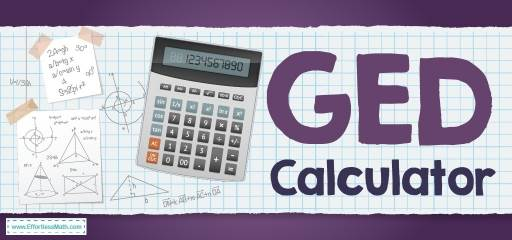# GED CalculatorIf you are preparing for the GED exam, you need to know whether a calculator can be used in mathematics or not. Join us to answer this question!

The GED math test has two parts. In the first part, which contains 5 questions, you are not allowed to use the calculator. For the second part, which contains 41 questions, you can use a calculator. The GED allows you to use the TI-30XS scientific calculator.

## The Absolute Best Book to Ace the GED Math Test

The TI-30XS is a scientific calculator, so it is a bit more difficult to use than a basic calculator. Don’t worry though. The GED test uses only the more basic functions of TI-30XS, and you do not need to learn advanced features to pass the test. You must learn how to use the TI-30XS before the test.

To get familiarized with the calculator, we recommend that you learn the following:

• Utilize the On button to reset the calculator or clear the memory.
• Use the All Clear and Clear buttons.
• Use the number keys and the basic operation keys for multiplication, addition, subtraction, and division.
• Find the equals, decimal point, and percent keys, and learn how and when each is used.
• Detect the Shift key and learn how and when it is used.
• Find the Fraction and Change Sign keys and how to use them.
• Find the Square Root and Square keys and learn when to use them.
• Enter problems using the correct sequence of operations.
• Find and use the keys for raising powers and creating exponents.

## GED FAQs:

Some common questions about the GED test:

### Can you use a calculator in a GED test?

Yes, you can bring the TI-30XS calculator with you to the test session. But if you take the GED test online, you can use the digital version on-screen calculator.

### What type of calculator is allowed on the GED?

You can only use the scientific calculator TI-30XS.

### When can you use a calculator on the GED?

You can use your calculator during the Science test, the Social Studies test, and the second part of the Math Reasoning test.

### How is the GED score calculated?

The scoring system of GED is complex, so a question is not always equal to one point. Some questions are fill in the blank or multiple choice, where you have to choose multiple answers, which means multiple points. The GED math section has 46 questions and a passing score of 145-164. According to the GED Testing Service, you need 60% -65% of your score to pass.

### How can I pass my GED math test?

Most people believe that the Math Reasoning part of the GED is the most challenging. And they do not take the GED because of math, but with the right guidance and hard work, you can easily pass the GED math.

### What is a calculator reference sheet?

The calculator reference sheet is provided to the test-takers to show the performance of the on-screen calculator, specifically on how to click the buttons in complex problems, such as calculation with fractions or the order of operations.

## More from Effortless Math for GED Test …

### Are you looking for a complete and FREE GED Math course to help you prepare for your GED Math test?

Check out our Ultimate GED Math Course.

Have a look at our comprehensive GED Math Worksheets to help you practice all GED Math concepts and prepare for the GED Math test.

### Want to review the most common GED Math formulas?

Here is our complete list of GED Math formulas.

Have a look at our Full-Length GED Math Practice Test and Free GED Math Practice Test.

## Have any questions about the GED Test?

### What people say about "GED Calculator - Effortless Math: We Help Students Learn to LOVE Mathematics"?

No one replied yet.

X
49% OFF

Limited time only!

Save Over 49%

SAVE \$34

It was \$69.99 now it is \$35.99